Using Functions

This section discusses how to:

• Configure modules for user functions.

• Define module parameters.

• Create user functions.

• Use built-in functions.

Pages Used to Define Functions

Page Name

Definition Name

Usage

Module Configuration

PF_FN_ENG_PNL

select EPM Foundation, then select EPM Setup, then select Common Definitions, then select System Objects, then select Module Configuration

Review configuration options or make changes.

Module Parameters

PF_FN_ENG_SEQ_PNL

select EPM Foundation, then select EPM Setup, then select Common Definitions, then select System Objects, then select Module Configuration, then select Module Parameters

View detailed information about the parameters for a module before you define a user function that is associated with a module.

User Functions

PF_FN_DEFN_PNL

• select EPM Foundation, then select Business Metadata, then select Constraint and Expressions, then select User Functions

• select Financial Services Industries, then select Risk-Weighted Capital Rules, then select Risk-Weighted Capital Rules, then select Function Definition, then select User Functions

Define functions that you can use with multiple analytic applications.

Built In Function

PF_FN_BIF_PNL

select EPM Foundation, then select EPM Setup, then select Common Definitions, then select System Objects, then select Built In Function

View predefined functions that are delivered with the system.

Module Configuration Page

Use the Module Configuration page (PF_FN_ENG_PNL) to review configuration options or make changes.

Image: Module Configuration page

This example illustrates the fields and controls on the Module Configuration page. You can find definitions for the fields and controls later on this page.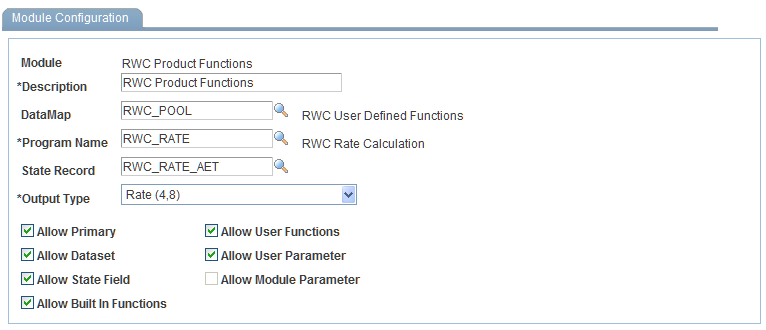Note: You should typically use the Module Configuration page only to verify information. You should not add or modify modules unless you have a thorough understanding of the system and the database tables that are affected. The only exception to this guideline concerns the Output Type field. This page displays pertinent module information that you may want to review before you define user formulas based on a specific module.

The DataMap code on the module page serves as the default DataMap displayed on the User Functions page. You can modify the DataMap on the User Functions page.

On the Module Configuration page, the DataMap Code designates the DataMap from which those who are defining user formulas are able to select. In the Program Name field, select the application engine that uses this module for its processing. If theAllow State Field check box is selected, the fields on the state record that is designated here are available to user formulas that are associated with this module. Output types define the output for user formulas that designate this module. You can change the output type. For example, if the output type is rate, and you want the result of a formula to be an amount, selectAmount from the drop-down list box. The options areAmount, Basis Points, Date, Integer, Rate, andString. TheAllow check boxes designate what types of data elements are allowed in user formulas that are associated with the module. For example, theAllow State Field check box, specifies that users defining formulas are able to use state fields in formulas that are associated with this module. The specific state record is designated in the top portion of this page. TheAllow Built In Functions andAllow User Functions check boxes designate functions that are provided with the system and functions that are defined through the User Functions page, respectively. TheAllow User Parameters andAllow Module Parameters check boxes are mutually exclusive. Module parameters are those that are defined on the Module Parameters page (which is automatically displayed if you selectAllow Module Parameters). User parameters are those that can be defined through the User Functions page by clicking the Input Parameters button at the top of the page (to the left of the Output Type field).

Module Parameters Page

Use the Module Parameters page (PF_FN_ENG_SEQ_PNL) to view detailed information about the parameters for a module before you define a user function that is associated with a module.

Use the Module Parameters page to verify information only. Do not add parameters.

If the Allow Module Parameters check box is selected on the Module Configuration page, the parameters that you define on this page are available for user-defined formulas that use the selected module.

User Functions Page

Use the User Functions page (PF_FN_DEFN_PNL) to define functions that you can use with multiple analytic applications.

Image: User Functions page

This example illustrates the fields and controls on the User Functions page. You can find definitions for the fields and controls later on this page.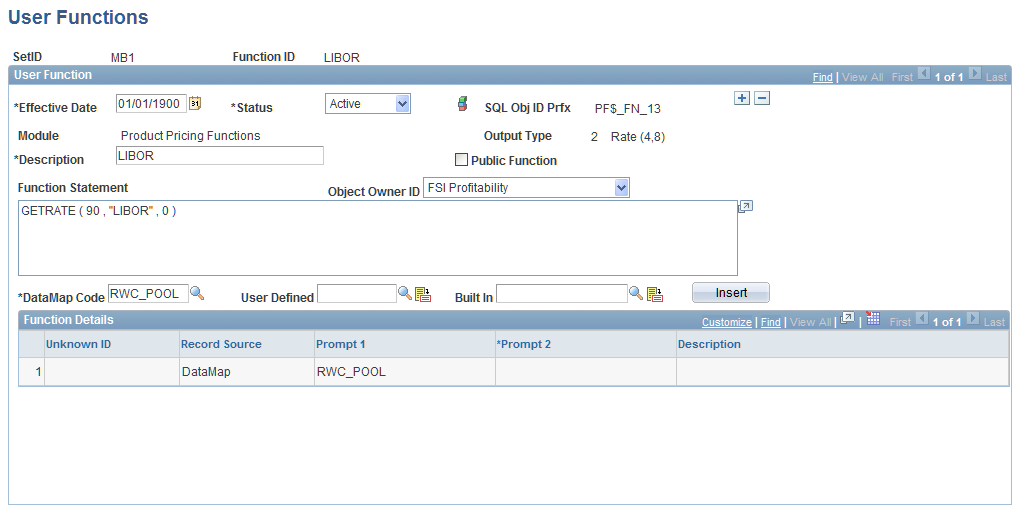To define user functions:

1. Enter an effective date and select a status.

2. The module that you select determines which data elements are available to this function.

3. To make this function available to all modules, select the Public Function check box.

Functions can call only other public user functions that use the same module. The check box is set by default to clear, which indicates a private function. This enables the function to use DataMaps (primary), DataSets, and state record fields. You can choose to make the function public, but public functions are not allowed to use DataMaps, DataSets, or state record fields.

Note: After a function is made public, you cannot change it to private.

4. Click the Insert button to insert a DataMap Code, User Defined, or Built In function into the grid.

The Output Type field on the User Functions page displays the input parameters that you specify.

5. After defining a parameter, you can use that parameter in the function if the Allow User Parameters check box is selected on the Module page for the module that is associated with this function.

Note: You should not change module definitions.

6. As you build the function, the function text appears in the Function box.

You can type directly in the Function box.

You can create parameters for the function and if the system does not recognize the parameter, the grid is populated with every token or unknown ID that the system does not recognize.

After you populate the grid, you select a datatype or record source for that ID.

The page is dynamic and the prompts vary according to your selection here.

The User Defined andBuilt In prompt look up public user defined functions and all built-in functions that exist in the system. After looking up the function ID, you can click the button to the right of the prompt to be taken to an informational page in the case of built-in functions. If you click the button for user defined it will transfer you modally to the user-defined function specified. After choosing either a built-in or user defined function, clickInsert to append the function at the end of the text in theFunction Statement box.

The function you insert defaults the parameters for each defined parameter in the function chosen.

Warning! The sum function works only with DataSets. Do not use it with a primary (DataMap) data element because it may return incorrect results.

Note: You cannot use the State within a public function.

Note: If you create an invalid function, the system displays an error message. However, the system still saves the function to preserve your work. Keep in mind that unless the system allows you to save the function without error, the function does not work.

Warning! The Built In Function page should be used to view the predefined functions only.

Using Built-In Functions

The following tables list the predefined functions that you can use when building custom functions. The following functions are reproduced under license from NAG, Inc. For detailed descriptions of the following functions, please consult NAG documentation.

NAG Functions

Function

Use

Mean (sample-col, wght-col)

Return the mean.

Std dev (sample-col, wght-col)

Standard deviation.

Std dev w

Standard deviation using weights.

Coeff of skewness (sample-col, wght-col)

Coefficient of skewness.

Coeff of skewness w.

Coefficient of skewness using weights.

Coeff of kurtosis (sample-col, wght-col)

Coefficient of kurtosis.

Coeff of kurtosis w

Coefficient of kurtosis using weights.

Lower hinge (input-col)

Lower hinge.

Upper hinge (input-col)

Upper hinge.

Median (input-col)

Median.

Binomial dist lt (n, p, k)

Binomial distribution, lower tail probability.

Binomial dist ut (n, p, k)

Binomial distribution, upper tail probability.

Binomial dist pt (n, p, k)

Binomial distribution, point probability.

poisson dist lt (rlamda, k)

Poisson distribution, lower tail probability.

Poisson dist ut (rlamda, k)

Poisson distribution, upper tail probability.

Poisson dist pt (rlamda, k)

Poisson distribution, point probability.

Hypergeom dist lt (n, l, m, k)

Hypergeometric distribution, lower tail probability.

Hypergeom dist ut (n, l, m, k)

Hypergeometric distribution, upper tail probability.

Hypergeom dist pt (n, l, m, k)

Hypergeometric distribution, point tail probability.

Deviates normal dist (p)

Deviate associated with given lower tail probability.

Rank scores sbr (input-col, rank)

Return element with this rank.

Rank scores sbe (input-col, elem)

Return rank of this element.

Normal scores sbr (input-col, rank).

Return element with this rank.

Normal scores sbe (input-col, elem)

Return rank of this element.

Prob normal lt

Standard distribution, lower tail probability.

Prob normal ttc

Standard distribution, two tail confidence interval probability.

Prob normal tts

Standard distribution, two tail significance level probability.

Prob normal ut

Standard distribution, upper tail probability.

Random beta n

Random beta, no repeat, meaning that the pseudo-random numbers generated from this function do not repeat.

Random beta r

The pseudo-random numbers that are output can repeat.

Rand cont uni abn

Random continuous uniform, taken over interval [a, b], no repeating.

Rand cont uni abr

Random continuous uniform, taken over interval [a, b], with repeating output.

Rand cont uni n

Random continuous uniform, no repeating output.

Rand cont uni r

Random continuous uniform, with repeating output.

Rand dis uniform n

Random discrete uniform, without repeating output.

Rand dis uniform r

Random discrete uniform, with repeat.

Rand exp n

Random number taken from exponential distribution without repeating output.

Rand exp r

Random number taken from exponential distribution with repeating output.

Rand gamma n

Random gamma, no repeat.

Rand gamma r

Random gamma, repeat.

Rand normal n

Random normal, no repeat.

Rand normal r

Random normal, repeat.

Ran permut vec n

Random permutation of an integer vector, no repeating output.

Ran permut vec r

Random permutation of an integer vector, with repeating output.

Ret dis binomial n

Return dis binomial, no repeat.

Ret dis binormial r

Return dis binomial, repeat.

Ret dis poisson n

Return dis poisson, no repeat.

Ret dis poisson r

Return dis poisson, no repeat.

savage scores sbr(*scores, *ties, input-col, rank)

Return element with this rank.

savage scores sbe(*scores, *ties, input-col, elem)

Return rank of this element.

Normal dist lt(*tail, x)

Lower tail probability.

Normal dist ut (*tail, x)

Upper tail probability.

Normal dist pt (*tail, x)

Point probability.

Student's-t lt (*tail, t, deg-of-freedom)

Lower tail probability.

Student's-t ut (*tail, t, deg-of-freedom)

Upper tail probability.

Student's-t tts (*tail, t, deg-of-freedom)

Two tail significance level probability.

Student's-t ttc (*tail, t, deg-of-freedom)

Two tail confidence level probability.

Prob chi sq lt (*tail, x, deg-of-freedom)

Lower tail probability.

Prob chi sq ut (*tail, x, deg-of-freedom)

Upper tail probability.

Prob f dist lt (*tail, f, df1, df2)

Lower tail probability.

Prob f dist ut (*tail, f, df1, df2)

Upper tail probability.

Prob beta dist lt (x, a, b, tol)

Lower tail probability.

Prob beta dist ut (x, a, b, tol)

Upper tail probability.

Prob beta dist pd (x, a, b, tol)

Probability density function.

Gamma dist lt (*tail, g, a, b)

Lower tail probability.

Gamma dist ut (*tail, g, a, b)

Upper tail probability.

Deviates normal lt (*tail, p)

Lower tail probability.

Deviates normal ut (*tail, p)

Upper tail probability.

Deviates normal tts (*tail, p)

Two tail significance level probability.

Deviates normal ttc (*tail, p)

Two tail confidence level probability.

Deviates students-t lt (*tail, p, df)

Lower tail probability.

Deviates students-t ut (*tail, p, df)

Upper tail probability.

Deviates students-t tts (*tail, p, df)

Two tail significance level probability.

Deviates students-t ttc (*tail, p, df)

Two tail confidence level probability.

Deviates chi sq (p, df)

Deviate associated with given lower tail probability.

Deviates f dist (p, df1, df2)

Deviate associated with given lower tail f probability.

Deviates beta (p, a, b, tol)

Deviate associated with given lower tail beta probability.

Deviates gamma dist (p, a, b, tol)

Deviate associated with given lower tail gamma probability.

Bivariate normal dist (x, y, rho)

Lower tail probability for bivariate Normal dist.

Beta dist lt

Beta dist, lower tail.

Beta dist pdf

Beta dist, probable density function.

Beta dist ut

Beta dist, upper tail.

Chi sq lt

Chi square, lower tail.

Chi sq ut

Chi square, upper tail.

Cumul normal

Cumulative normal distribution.

Erf

Error function.

Erfc

Complementary error function.

F dist lt

F dist, lower tail.

F dis ut

F dist, upper tail.

Gamma

Gamma function.

Getchardata

Get a character value from a column.

Getdatedata

Get a date value from a column.

Getnumericdata

Get numeric data from a column.

Getrate

Get yield rate.

This function is used exclusively by the Product Pricing Module.

Inc gamma p

Incomplete gamma function.

Inc gamma q

Incomplete gamma function.

Mean weighted

Mean, weighted.

Tsa auto corr coef

TSA auto correlation.

Tsa auto corr mean

TSA auto correlation mean.

Tsa auto corr parm

TSA auto correlation parameter.

Tsa auto corr part

TSA auto correlation partial.

Tsa auto corr pred

TSA auto correlation predictor.

Tsa auto corr stat

TSA auto correlation stat.

Tsa auto corr var

TSA auto correlation variance.

Other Predefined Functions

Function

Use

Average (col)

Returns the average of a column.

Count (col)

Returns the number of columns.

Earliest (col)

Earliest of all periods.

Latest (col)

Latest of all periods.

Max (col)

Returns the maximum value of the set.

Min (col)

Returns the minimum value of the set.

MaxVal (x, y)

If x > y return x else y.

MinVal (x, y)

If x < y return x else y.

Sum (col)

Returns the sum of the set.

Works only with DataSets. Do not use with a Primary (DataMap) Data Element.

Beginning of month (date_from)

Beginning of date's month.

Beginning of year (date_from)

Beginning of date's year.

End of month (date_from)

End of date's month.

End of year (date_from)

End of date's year.

Round (expression, factor)

Percent round.

Substring (string, start, length)

Substring of a string.

TrimSubStr (string, start, length)

Substring without trailing blanks.

Truncate (expression, factor)

Truncate a numeric value.

Numeric value to truncate.

Number of decimal places to truncate to.

ln (x)

Natural log.

log10 (x)

Log base 10.

Square Root (x)

Square root.

Cos (x)

Cosine.

Sin (x)

Sine.

Tan (x)

Tangent.

Absolute (x)

Absolute value of x.

Date (string)

Convert string to date.

RunDate ( )

Run date of the job.

Uses the system date.

Frequency (col, elem)

Frequency of a specified element in a column.

Product (col)

Product of series of elements.

MSE (col, *n, degrees-of-freedom).

Mean squared error.

Mean absolute deviation.

RWC_BRW1

For BASEL Accord calculations. This function calculates the risk weight (RWc) for corporate exposures, according to the formula specified following this table.

RWC_BRW2

Calculation of an exposure's risk weight (RWc) with maturity adjustment for corporate exposures, according to the formula specified following this table.

RWC_BCR1

Calculation of the capital requirement K with maturity adjustment, according to the formula specified following this table.

RWC_BCR2

Calculation of the capital requirement K with firm-size adjustment, according to the formula specified following this table.

RWC_BCR3

Calculation of the capital requirement K for residential mortgage exposures, according to the formula specified following this table.

RWC_BCR4

Calculation of the capital requirement K for qualifying revolving exposures, according to the formula specified following this table.

RWC_BCR5

Calculation of the capital requirement K for other retail exposures, according to the formula specified following this table.

RWC_BRW1

Formula: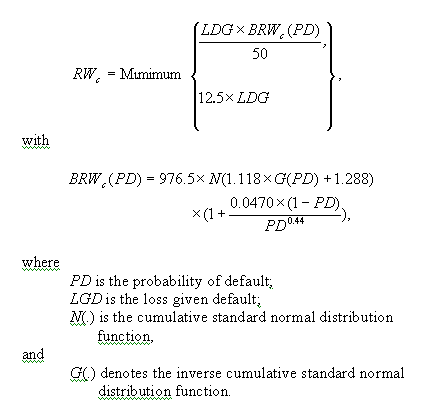Calling Sequence: RWC = RWC_BRW1(PD,LGD)

RWC_BRW2

Formula: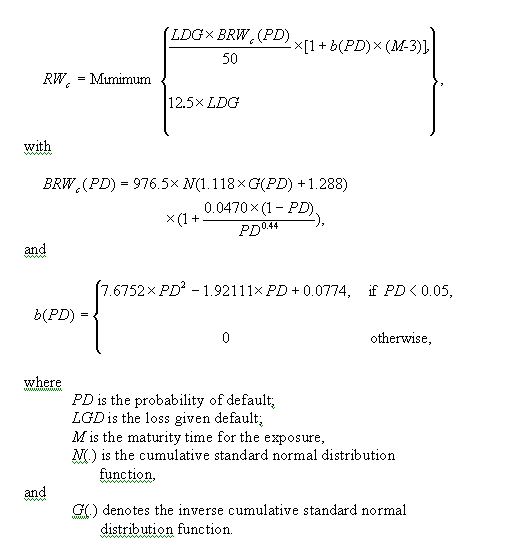Calling Sequence: RWC = RWC_BRW2(PD,LGD,M)

RWC_BCR1

Formula: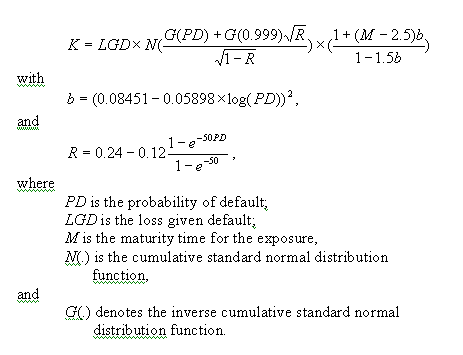Calling Sequence: RWC_BCR1(PD,LGD,M)

RWC_BCR2

Formula: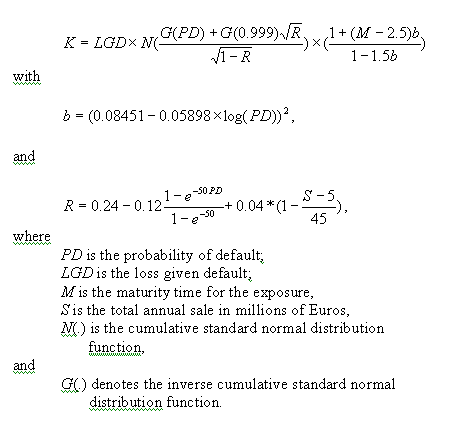Calling Sequence: K = RWC_BCR2(PD,LGD,M,S)

RWC_BCR3

Formula: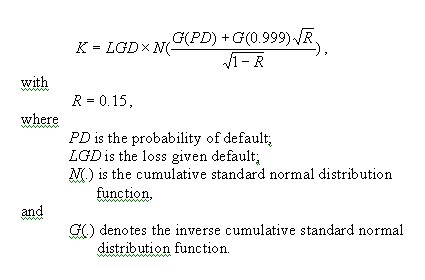Calling Sequence: K = RWC_BCR3(PD,LGD)

RWC_BCR4

Formula: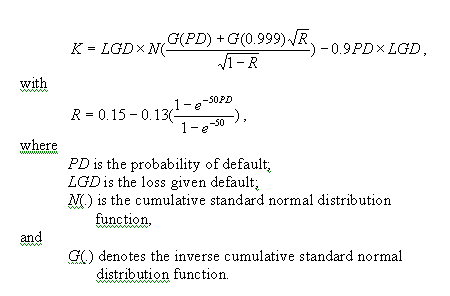Calling Sequence: K = RWC_BCR4(PD,LGD)

RWC_BCR5

Formula:Calling Sequence: K = RWC_BCR5(PD,LGD)